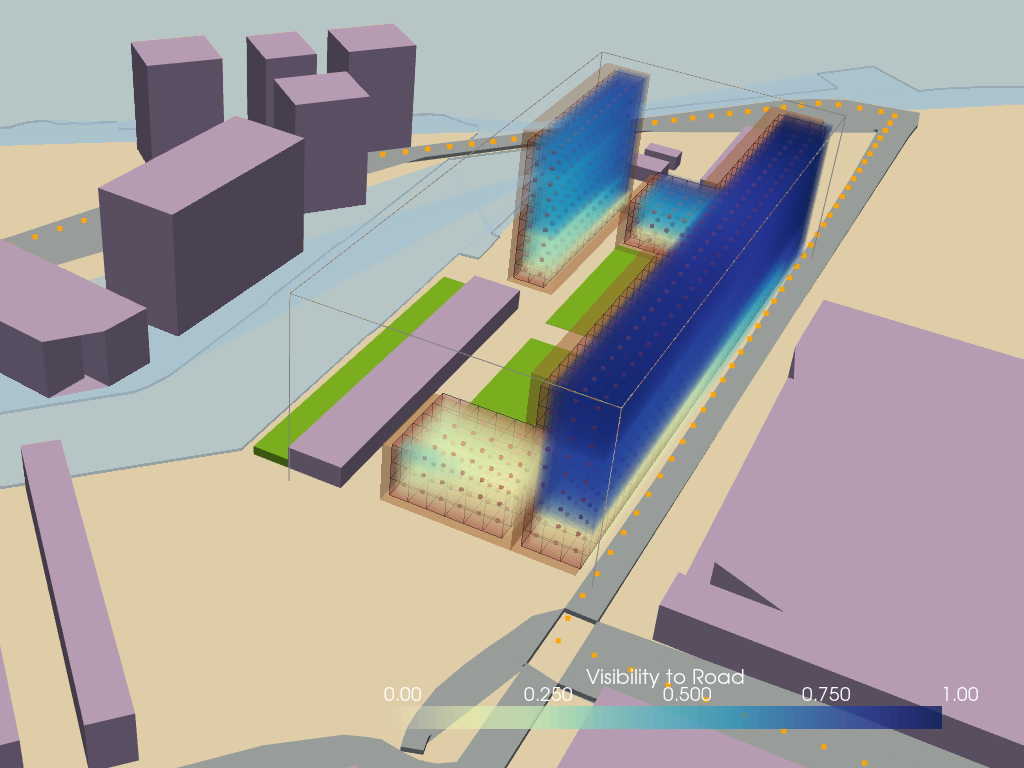# Enviornment Simulation Lattices⌗

The simulations which were performed were based on the specific case considered in the project. The idea at this stage is not to generate a highly accurate simulation models, but simulations which are good enough for decision making. The generation of highly accurate simulations is also not possible at this stage since the material properties and the geometry of the various building elements is not defined yet.

The simulations are broadly divided into two types. The first one is based on finding `Eucledian distances` and the second one is based on `Ray obstruction` .

# Base voxelization⌗

The initial step before calculations of simulation lattices was to separate the 4 masses into separate lattices for generating values locally for the individual masses. The voxelated lattice of the massing as derived from Massing Problem is a numpy n-dimensional array consisting of ones and zeroes. Ones represents the voxels inside the 3d object which are available for occupation and zero represents the exterior voxels which are not relevant to the simulations.The pseudo-code for voxelation is as follows.

``````import os
import topogenesis as tg
import pyvista as pv
import trimesh as tm
import numpy as np

# Input Mesh

# load the mesh from file
# Check if the mesh is watertight
print(mesh.is_watertight)

# convert trimesh to pv_mesh
def tri_to_pv(tri_mesh):
faces = np.pad(tri_mesh.faces, ((0, 0),(1,0)), 'constant', constant_values=3)
pv_mesh = pv.PolyData(tri_mesh.vertices, faces)
return pv_mesh

#Voxelize the mesh

# initialize the base lattice
base_lattice = tg.lattice(mesh.bounds, unit=unit, default_value=1, dtype=int)

# check which voxel centroids is inside the mesh
interior_condition = mesh.contains(base_lattice.centroids)

# reshape the interior condition to the shape of the base_lattice
interior_array = interior_condition.reshape(base_lattice.shape)

# convert the interior array into a lattice
interior_lattice = tg.to_lattice(interior_array, base_lattice.minbound, base_lattice.unit)

# Save to Csv
csv_path = os.path.relpath('voxelized_envelope_6m_voxel_size.csv')
interior_lattice.to_csv(csv_path)

``````

The binder link to the code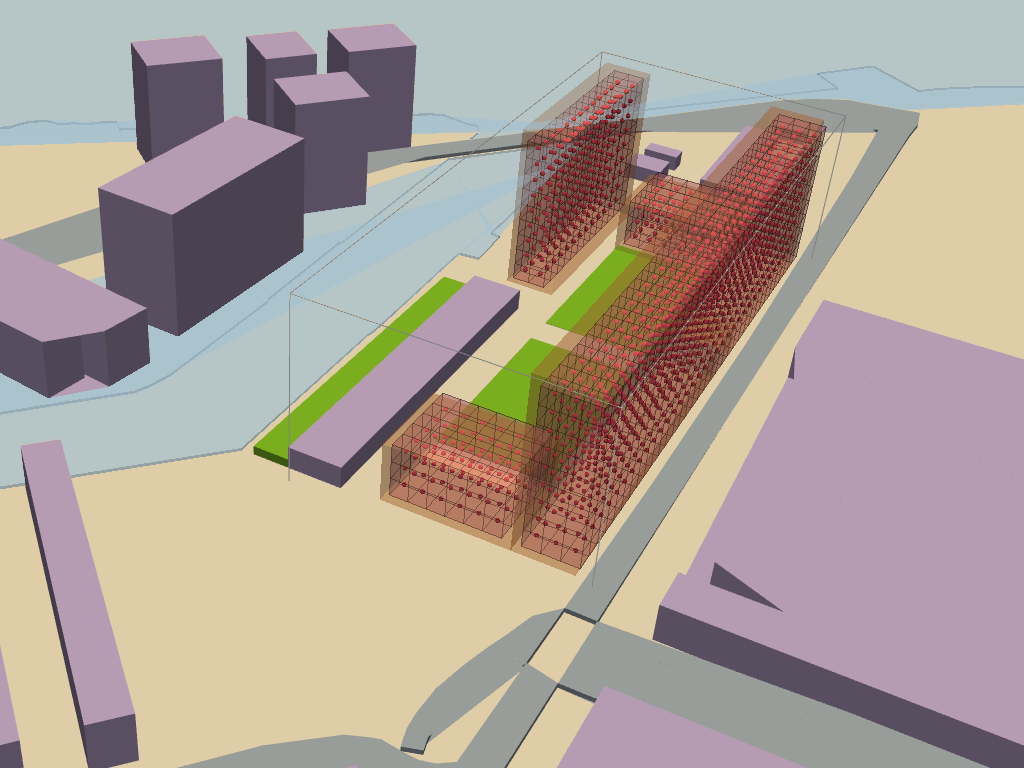Voxelised Base

# Eucledian Distance based simulations⌗

## Quiteness lattices⌗

The Quiteness Lattice is a measure of the sound pressure recieved at each of the locations in the mass or the voxelated grid.The calculations related to the sound insulation are complicated and require a lot of data with respect to the material qualities of the building the sound pressure at the source of sound etc.The general equation for airborne sound insulation of a facade is given as:

The distance from the source of the sound to the room is critical in this calculation and there can be a 6Db reduction in the sound pressure level with doubling of the distance from the source.This relation can be easily calculated, and will provide a good estimate for decision making regarding the quietness of the voxel with respect to the source of sound.The code for calculating the same would be as follows:

``````# Upload base voxel grid

# Find the voxel Eucledian coordinates
# convert to lattice
init_lattice = envelope_lattice +1
availability_lattice_voxels = tg.to_lattice(init_lattice, init_lattice)
voxel_coordinates= availability_lattice_voxels.centroids
flattened_lattice = envelope_lattice.flatten()

# Find distances for Quiteness
# The distance from each source of sound is calculated and the minimum distance from them is choosen

Eucledian_distance = sc.spatial.distance.cdist(Noise_sources,voxel_coordinates)
noise_from_each_source = Eucledian_distance.T
Average_quiteness_indexing= np.argmin(noise_from_each_source, axis=1)
Average_quiteness_values = []

for branch,index in zip(noise_from_each_source,Average_quiteness_indexing):
Average_quiteness_values.append(branch[index])

Quiteeness_lattice_padded= np.array([num if boolean else 0 for boolean, num in zip(flattened_lattice, cycle(Average_quiteness_values))])

Quiteness_lattice =tg.to_lattice(Quiteness_lattice_np, Quiteness_lattice_np.shape)

``````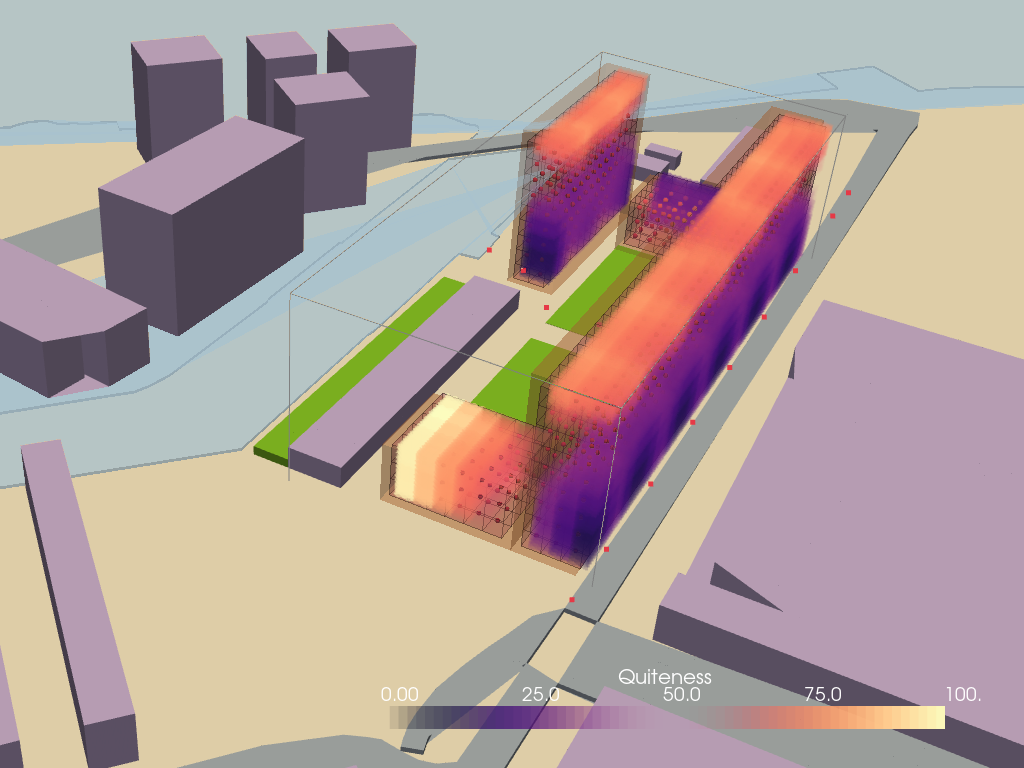## Closeness to the Facades lattices⌗

The facade closeness lattice determines the distance of the voxels in an lattice with respect to the outermost voxels in a specified direction. The calculations for the euclidean distances or the closeness was done for all the facades in the four cardinal directions and to the terrace and the ground for all the masses.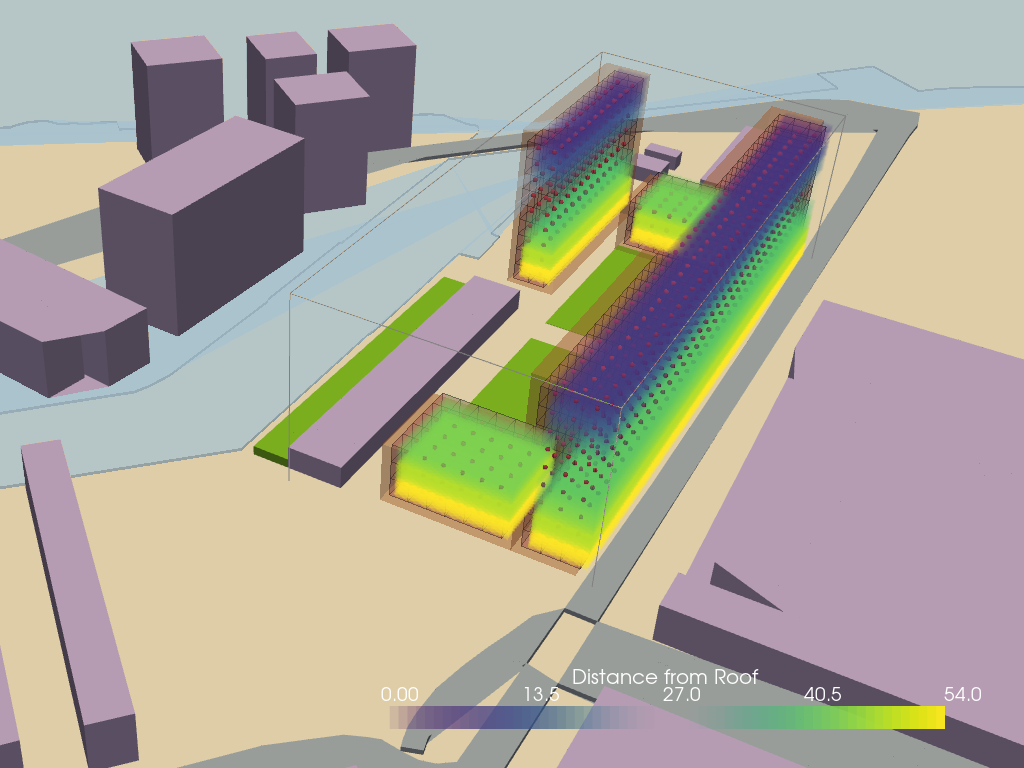Distance from the Roof Lattice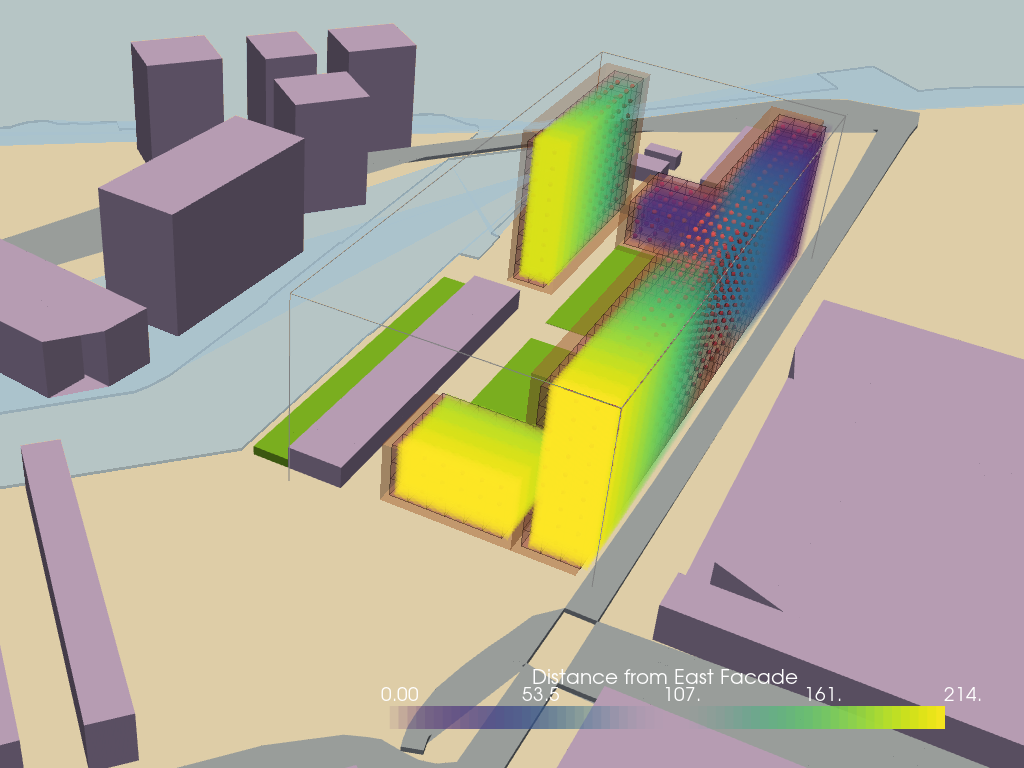Distance from the East facade Lattice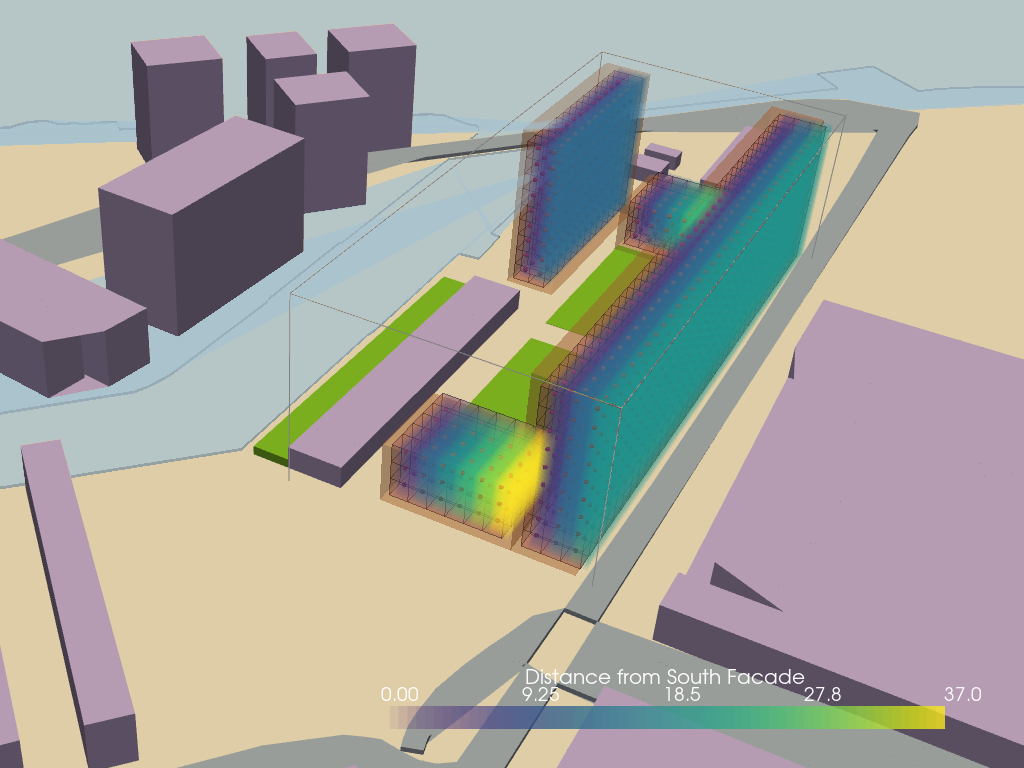Distance from the South Facade Lattice

The binder link to the code for generating distance based lattices# Mesh Intersection based simulations⌗

## Sun access lattice⌗

Access to daylight is critical for a good quality of indoor environment and has shown links to productivity and wellbeing. Daylight analysis is generally done in a later stage of design process where the internal layout of spaces and the geometry and sizes of the openings in the spaces are already designed. The material properties of the elements used in facades and inside the spaces themselves also play a big role in determining the amount of daylight received inside a space. At an early design stage the access to sun received by the mass is also a good enough metric to calculate the daylight factor for the building. This can be calculated by calculating the `Vertical sky component (VSC)` Vertical sky component is the amount of sky which is visible from a point of reference considering the obstructions in the surroundings. That area of visible sky is expressed as a percentage of an unobstructed hemisphere of sky, and, therefore, represents the amount of daylight available for that particular window. It does not consider the size of the room or the number of windows it only calculates the access to the sky.

The pseudocode for the calculation would be as follows:

``````# Extract centorids of voxels
Centroids_of_voxels = tg.centroids(base_lattice)

# Extract Sun locations
Import epw file of the location

Selected_sun_position_hours = [array of selected hours]
# Use the sun hours as indices to extact locations of the sun positions from the weather file

Selected_sun_positions = all positions[Selected_sun_position_hours]

#Shoot rays from the voxel centroids and sun positions
Ray_sources = Selected_sun_positions
Ray_directions = Centroids_of_voxels

# Calculate intersections with context mesh
Building_id , ray_id = Context_mesh_for_building_mesh.ray.intersects_id(ray_origins=Ray_sources, ray_directions= Ray_directions, multiple_hits=False)

# Calculate the percentage of hits for all the voxel positions which will be the access to the sun in percentage

Sun_Access_Lattice = % of hits for all the rays on each voxel position

``````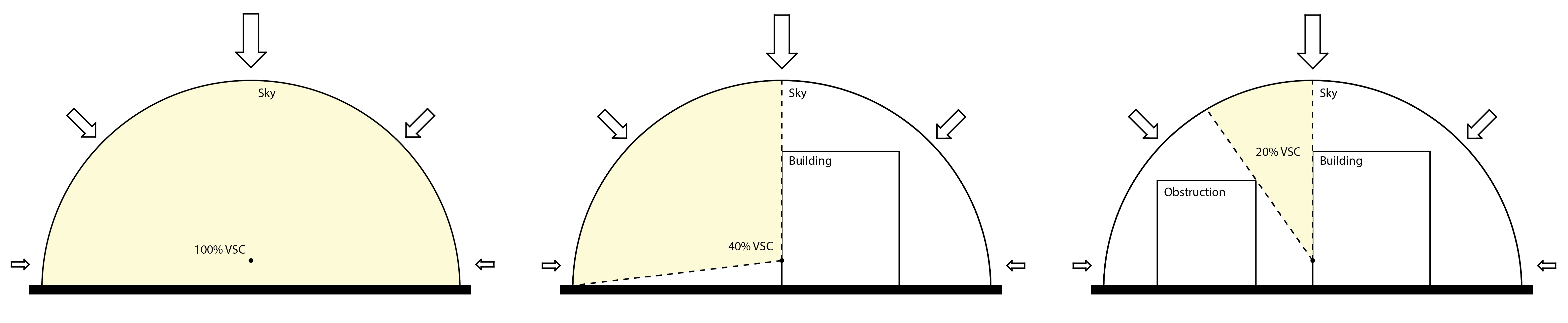Vertical Sky Component diagram image source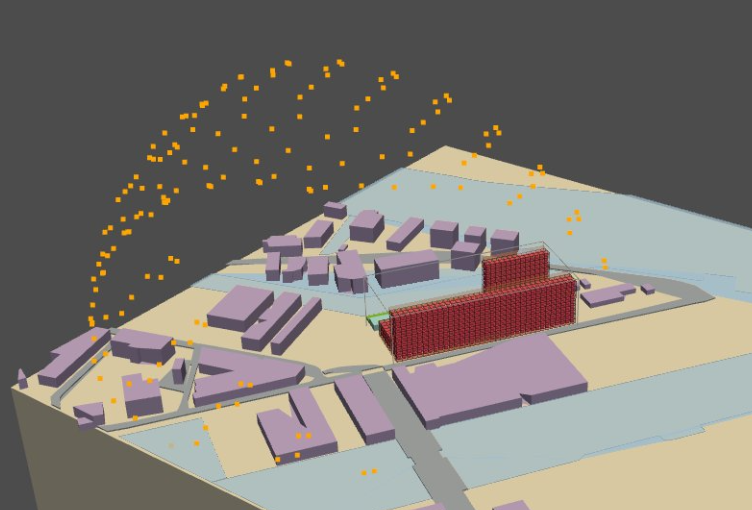Sources of Sun

The library Ladybug tools was used to generate the sun positions based on the location for the particular site.These locations were used to create sun vectors from which rays were shot towards the centroids of the voxels. The intersection with the context was checked using the library trimesh and the percentage of non-intersected rays was calculated which is the vertical sky component.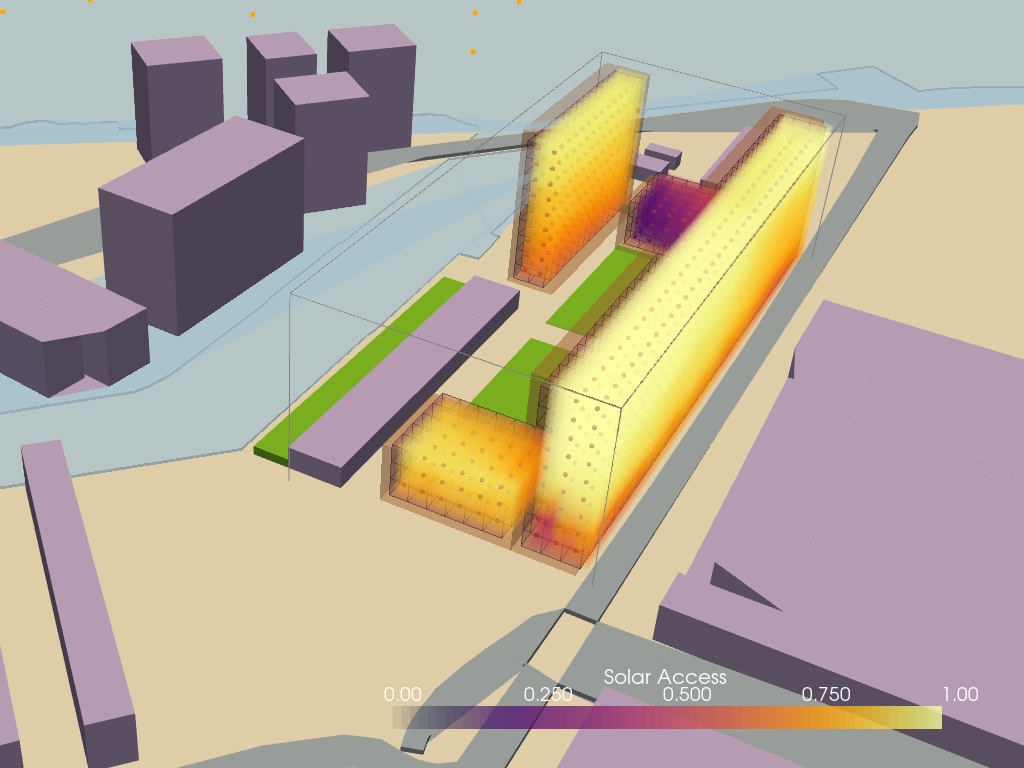Sun Access Lattice

The binder link to the code for generating solar access lattice## Visibility lattice⌗

A visibility analysis is important to consider the compatibility of a building with its surroundings. The visibility of a building from different viewpoints like main streets public areas or other buildings becomes critical to location of certain functions which thrive on a better visibility like retail shops and restaurants. The degree of privacy can also be determined based on the direct visibility of a space from a point.

The characteristics of building locations, building height arrangements, and other circumstances need to be considered in a study of visibility analysis. Visibility studies in a two-dimensional representation have been utilized for a long time for landscape, architectural, and urban studies to calculate the view of a structure or element in the spatial environment. However, a 3D study becomes more comprehensive and accurate to determine the visibility.

The approach taken to calculate the visibility is similar to the sun access lattice where percentage of intersection is calculated to determine the visibility to a point of interest. For the particular case at Buiksloterham two visibility lattices are generated one which calculates the visibility towards the IJ and the other one calculates the visibility from the road.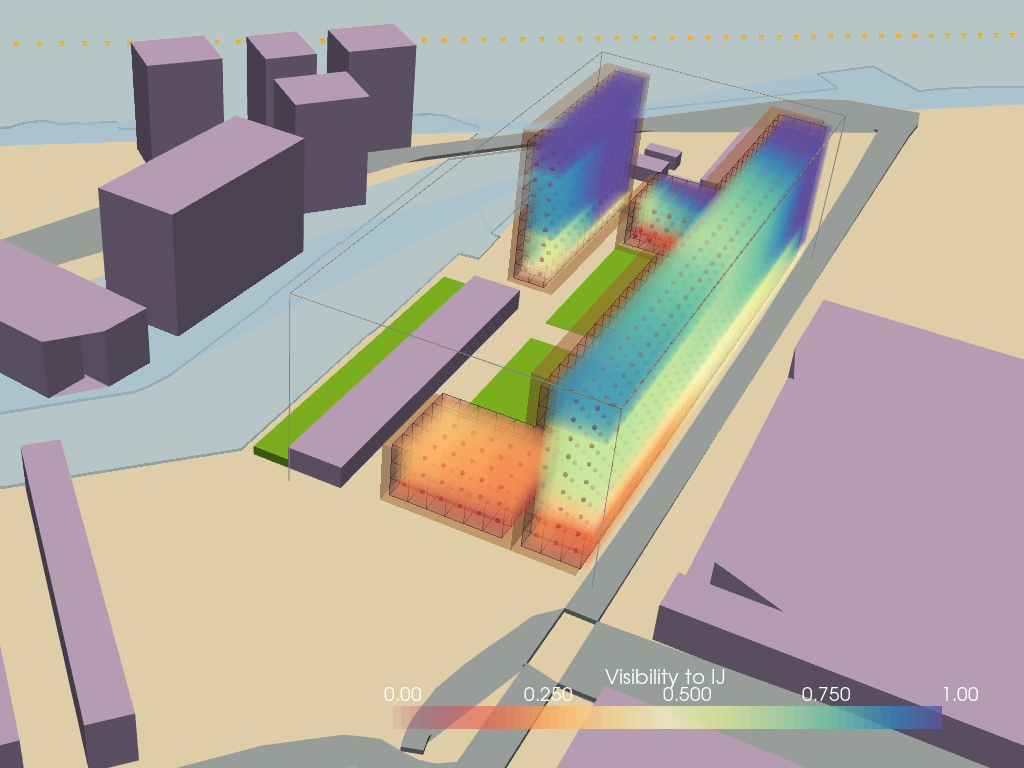Visibility to the IJ Lattice